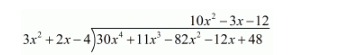# Divide 30x4 + 11x3 − 82x2 − 12x + 48`
Question:

Divide 30x4 + 11x3 − 82x2 − 12x + 48 by (3x2 + 2x − 4) and verify the result by division algorithm.

Solution:

Here we have to divide $30 x^{4}+11 x^{3}-82 x^{2}-12 x+48$ by $3 x^{2}+2 x-4$.$30 x^{4}+20 x^{3}-40 x^{2}$

$-9 x^{3}-42 x^{2}-12 x+48$

$-9 x^{3}-6 x^{2}+12 x$

$-36 x^{2}-24 x+48$

$-36 x^{2}-24 x+48$

According to division algorithm, Dividend = Divisor × Quotient + Remainder.

This can be verified as,

Divisor × Quotient + Remainder

$\left(3 x^{2}+2 x-4\right) \times\left(10 x^{2}-3 x-12\right)$

$=30 x^{4}-9 x^{3}-36 x^{2}+20 x^{3}-6 x^{2}-24 x-40 x^{2}+12 x+48$

$=30 x^{4}+11 x^{3}-82 x^{2}-12 x+48$

$=$ Dividend